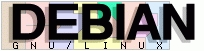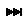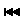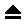GNU Info

Info Node: (elisp)Function Indirection

#(elisp)Function Indirection

Next:Function Forms Prev:Classifying Lists Up:Forms
Enter node , (file) or (file)node
```
Symbol Function Indirection
---------------------------

If the first element of the list is a symbol then evaluation examines
the symbol's function cell, and uses its contents instead of the
original symbol.  If the contents are another symbol, this process,
called "symbol function indirection", is repeated until it obtains a
non-symbol.  Note: Function Names, for more information about using a
symbol as a name for a function stored in the function cell of the
symbol.

One possible consequence of this process is an infinite loop, in the
event that a symbol's function cell refers to the same symbol.  Or a
symbol may have a void function cell, in which case the subroutine
`symbol-function' signals a `void-function' error.  But if neither of
these things happens, we eventually obtain a non-symbol, which ought to
be a function or other suitable object.

More precisely, we should now have a Lisp function (a lambda
expression), a byte-code function, a primitive function, a Lisp macro, a
special form, or an autoload object.  Each of these types is a case
described in one of the following sections.  If the object is not one of
these types, the error `invalid-function' is signaled.

The following example illustrates the symbol indirection process.  We
use `fset' to set the function cell of a symbol and `symbol-function'
to get the function cell contents (Note: Function Cells).
Specifically, we store the symbol `car' into the function cell of
`first', and the symbol `first' into the function cell of `erste'.

;; Build this function cell linkage:
;;   -------------       -----        -------        -------
;;  | #<subr car> | <-- | car |  <-- | first |  <-- | erste |
;;   -------------       -----        -------        -------

(symbol-function 'car)
=> #<subr car>
(fset 'first 'car)
=> car
(fset 'erste 'first)
=> first
(erste '(1 2 3))   ; Call the function referenced by `erste'.
=> 1

By contrast, the following example calls a function without any
symbol function indirection, because the first element is an anonymous
Lisp function, not a symbol.

((lambda (arg) (erste arg))
'(1 2 3))
=> 1

Executing the function itself evaluates its body; this does involve
symbol function indirection when calling `erste'.

The built-in function `indirect-function' provides an easy way to
perform symbol function indirection explicitly.

- Function: indirect-function function
This function returns the meaning of FUNCTION as a function.  If
FUNCTION is a symbol, then it finds FUNCTION's function definition
and starts over with that value.  If FUNCTION is not a symbol,
then it returns FUNCTION itself.

Here is how you could define `indirect-function' in Lisp:

(defun indirect-function (function)
(if (symbolp function)
(indirect-function (symbol-function function))
function))

```

automatically generated by info2www version 1.2.2.9